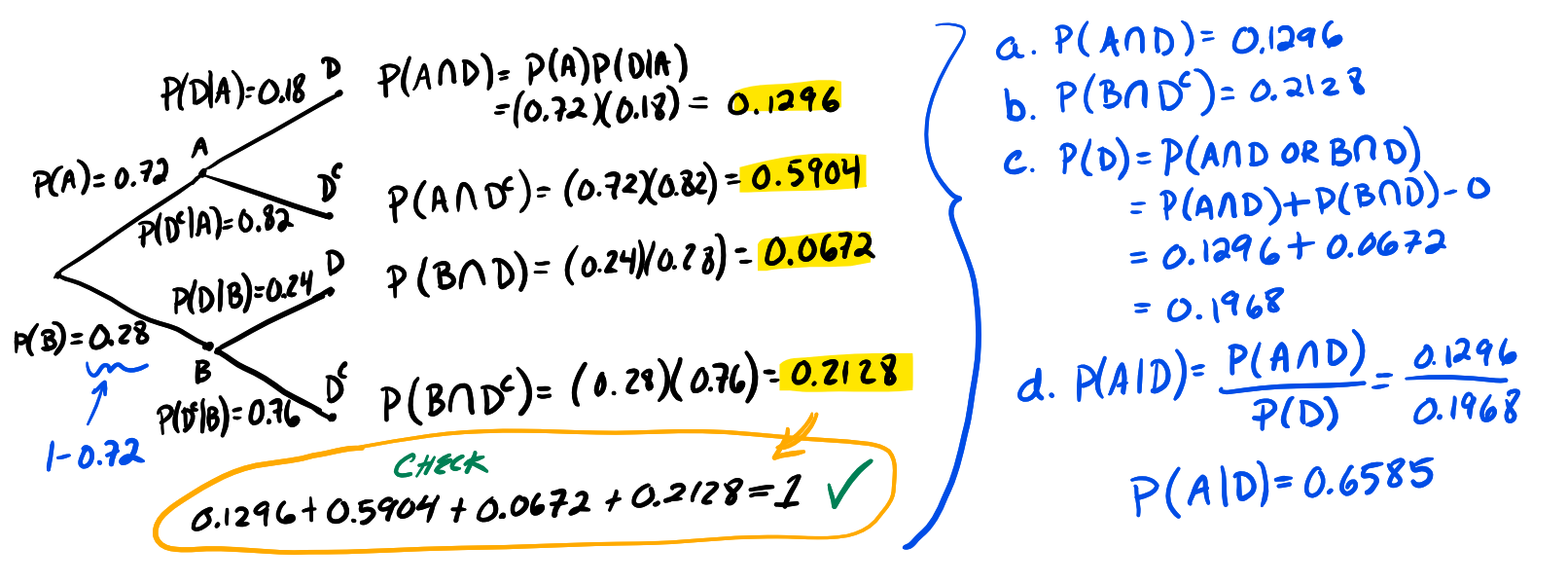1
2
3
4
5
#

Bayes' Theorem

Overview

If the P(B) > 0, then the following equation is a mathematical representation of Bayes' theorem:

$$P(A|B) = \frac{P(A) P(B|A)} { P(B)}$$

In short, if P(B | A) is known, then Bayes' Theorem is ideally suited to find the conditional probability of finding the event P(A | B). The simplest way to familiarize yourself with Bayes' Theorem is to jump in and do hands-on examples and exercises, as those provided below.

Examples

1. At Jackson High School, there are two types of math classes, regular or advanced. The probability a student is an advanced math class is 0.2. For regular classes, the probability a student loves math is 0.4. For advanced classes, the probability a student loves math is 0.6. Given this information:
a. Construct a tree diagram. Determine the outcomes of the sample space and then assign probabilities to each outcome.
b. Calculate the probability a randomly chosen student is in an advanced class and does not love math.
c. Calculate the probability a randomly chosen student loves math.
d. Calculate the probability a student is from an advanced math class given that they don't like math.

2. A cell phone factory builds phones using two machines, they are labeled simply as Machine A and Machine B. Production records show that 72% of cell phones are produced on Machine A. Furthermore, 18% of all phones built using machine A are defective and 24% of all phones built on machine B are defective. Given this information:
a. Calculate the probability that a random cell phone was produced on Machine A and is defective
b. Calculate the probability that a random cell phone was produced on Machine B and is not defective.
c. Calculate the probability that a random cell phone was produced is defective.
d. Using Bayes' Theorem, calculate the probability that a cell phone was produced on Machine A given that it was found to be defective.